## please help!! A baseball cannon fires balls at an initial speed of 29 m/s. The cannon is tilted at a 52o angle. (a) How lo

Question

A baseball cannon fires balls at an initial speed of 29 m/s. The cannon is tilted at a 52o angle.
(a) How long could one of these balls stay in the air ?
(b) How far from the cannon, will the ball hit the ground ?
(c) What will be ball’s maximum altitude ?

in progress 0
6 months 2021-08-27T06:17:47+00:00 1 Answers 1 views 0

(a) The time the ball stays in the air is approximately 4.66 seconds

(b) The distance from the cannon at which the ball will hit the ground is approximately 83.18 meters

(c) The maximum altitude of the ball is approximately 26.62 m

Explanation:

The given parameters of the question are;

The initial velocity at which a baseball cannon fires balls, u = 29 m/s

The angle at which the cannon is tilted, θ = 52°

(a) The time duration the ball stays in the air is given by the time of flight of the projected ball as follows;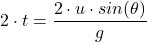Where;

t = The time it takes the baseball to reach maximum height

2·t = The total time of flight = The time the ball stays in the air

θ = The angle at which the ball is tilted = 52°

g = The acceleration due to gravity ≈ 9.81 m/s²

u = The initial velocity = 29 m/s

Therefore, we have;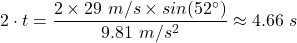The time the ball stays in the air, 2·t ≈ 4.66 seconds

(b) The distance from the cannon at which the ball will hit the ground is given by the horizontal range, ‘R’, of the projectile as follows;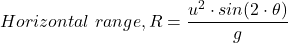∴ The distance from the cannon at which the ball will hit the ground = R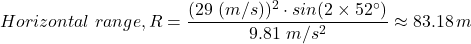The distance from the cannon at which the ball will hit the ground = R ≈ 83.18 meters

(c) The maximum altitude of the ball is equal to the maximum height reached by the ball, H, which is given as follows;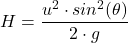Therefore, we have;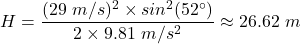The maximum altitude of the ball ≈ 26.62 m